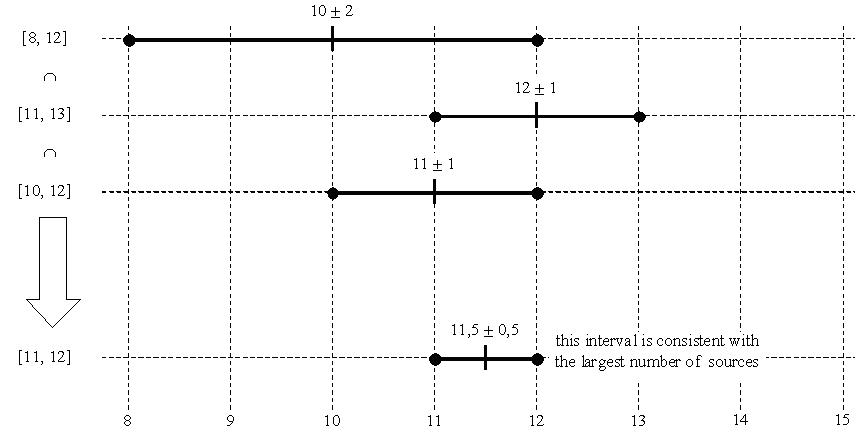# Sntpd开发日志2 Marzullo算法1. s.poll 的区间设置得过长，时钟一旦偏移，要花很长的时间去校正
2. 由于95%置信区间的设定，导致时钟源总是变化，因此总有跳变

https://en.wikipedia.org/wiki/Marzullo%27s_algorithm``````# iset 即区间拆分之后的数值
func (s *Service) marzullo(iset []Interset) (surviors []*Peer) {
sort.Sort(byOffset(iset))
nlist := len(iset) / 2
nl2 := len(iset)
var n int
low := time.Hour
high := -time.Hour
for allow := 0; 2*allow < nlist; allow++ {
n = 0
for _, set := range iset {
low = set.val
n -= set.typE
if n >= nlist-allow {
break
}
}
n = 0
for j := nl2 - 1; j > 0; j-- {
high = iset[j].val
n += iset[j].typE
if n >= nlist-allow {
break
}
}
if high > low {
break
}
}
var p *Peer
for i := 0; i < len(iset); i += 2 {
p = iset[i].peer
if high <= low || p.offset+p.rootDistance < low || p.offset-p.rootDistance > high {
continue
}
surviors = append(surviors, p)
}
return
}
``````